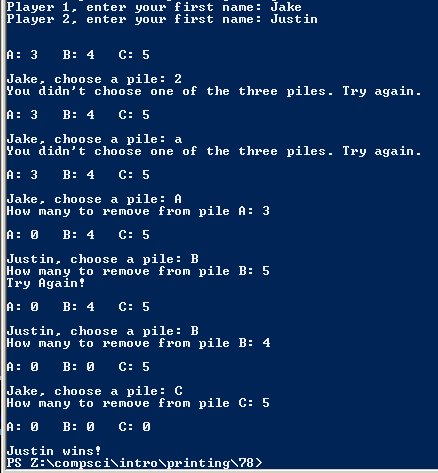PROGRAMMING AND CODE

# Project

## Code

```/// Name: Justin Li
/// Period: 7
/// Program Name: Nim
/// File Name: Nim.java
/// Date Completed: 2/14/16

import java.util.Scanner;

public class Nim {

public static void main(String[] args) {

Scanner keyboard = new Scanner(System.in);

String a1, a2, ac;
int p1, p2, p3, pc, n;

n = 0;
p1 = 3;
p2 = 4;
p3 = 5;

System.out.print("Player 1, enter your first name: ");
a1 = keyboard.next();

System.out.print("Player 2, enter your first name: ");
a2 = keyboard.next();

System.out.println("\n\nA: " + p1 + "   B: " + p2 + "   C: " + p3);

while (p1 + p2 + p3 != 0) {

if (n % 2 == 0) {

System.out.print("\n" + a1 + ", choose a pile: ");
ac = keyboard.next();

if (!ac.equals("A") && !ac.equals("B") && !ac.equals("C")) {
System.out.println("You didn't choose one of the three piles. Try again.");
n--;
}

else {
System.out.print("How many to remove from pile " + ac + ": ");
pc = keyboard.nextInt();

if (ac.equals("A")) {
p1 = p1 - pc;
if (p1 < 0 || pc <= 0) {
System.out.println("You are trying to cheat, try again.");
p1 = p1 + pc;
n--;
}
}
else if (ac.equals("B")) {
p2 = p2 - pc;
if (p2 < 0 || pc <= 0) {
System.out.println("You are trying to cheat, try again.");
p2 = p2 + pc;
n--;
}
}
else if (ac.equals("C")) {
p3 = p3 - pc;
if (p3 < 0 || pc <= 0) {
System.out.println("You are trying to cheat, try again.");
p3 = p3 + pc;
n--;
}
}
}
n++;
}

else if (n % 2 == 1) {

System.out.print("\n" + a2 + ", choose a pile: ");
ac = keyboard.next();

if (!ac.equals("A") && !ac.equals("B") && !ac.equals("C")) {
System.out.println("You didn't choose one of the three piles. Try again.");
n--;
}

else {
System.out.print("How many to remove from pile " + ac + ": ");
pc = keyboard.nextInt();

if (ac.equals("A")) {
p1 = p1 - pc;
if (p1 < 0 || pc <= 0) {
System.out.println("Try Again!");
p1 = p1 + pc;
n--;
}
}
else if (ac.equals("B")) {
p2 = p2 - pc;
if (p2 < 0 || pc <= 0) {
System.out.println("Try Again!");
p2 = p2 + pc;
n--;
}
}
else if (ac.equals("C")) {
p3 = p3 - pc;
if (p3 < 0 || pc <= 0) {
System.out.println("Try Again!");
p3 = p3 + pc;
n--;
}
}
}
n++;
}

System.out.println("\nA: " + p1 + "   B: " + p2 + "   C: " + p3);

}

if (n % 2 == 0)
System.out.println("\n" + a1 + " wins!");
else if (n % 2 == 1)
System.out.println("\n" + a2 + " wins!");
}
}
```

### Picture of the output